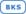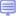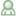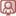# SAS/ETS

Titlul Nivel Formatul cursurilor
Stationarity Testing and Other Time Series TopicsThis course addresses a basic question in time series modeling and forecasting: whether a time series is nonstationary. This question is addressed by the unit root tests. One of the most common tests, the Dickey-Fuller test, is discussed in this lecture.

3 Nivel mediuEstablishing Causal Inferences: Propensity Score Matching, Heckman's Two-Stage Model, Interrupted Time Series, and Regression Discontinuity ModelsThis course introduces some methods commonly used in program evaluation and real-world effectiveness studies, including two-stage modeling, interrupted time-series, regression discontinuity, and propensity score matching. These methods help address questions such as: Which medicine is more effective in the real world? Did an advertising program have an impact on sales? More generally, are the changes in outcomes causally related to the program being run?

3 Nivel mediuTime Series Modeling Essentials
This course discusses the fundamentals of modeling time series data. The course focuses on the applied use of the three main model types used to analyze univariate time series: exponential smoothing, autoregressive integrated moving average with exogenous variables (ARIMAX), and unobserved components (UCM).

The e-learning format of this course includes Virtual Lab time to practice.

3 Nivel mediuStatistics 2: ANOVA and Regression
Acest curs tratează analiza variabilelor explicate continue și a variabilelor discrete. Sunt prezentate regresia liniară, regresia Poisson, regresia binomială negativă, regresia gamma, analiza varianței, regresia liniară cu variabile dihotomice, analiza covarianței și modele ANOVA mixte.

3 Nivel mediuForecasting Using SAS Software: A Programming Approach
This course teaches analysts how to use SAS/ETS software to diagnose systematic variation in data collected over time, create forecast models to capture the systematic variation, evaluate a given forecast model for goodness-of-fit and accuracy, and forecast future values using the model. Topics include Box-Jenkins ARIMA models, dynamic regression models, and exponential smoothing models.

4 ExpertState Space Modeling Essentials Using the SSM Procedure in SAS/ETS
This course covers the fundamentals of building and applying state space models using the SSM procedure (SAS/ETS). Students are presented with an overview of the model and learn advantages of the State Space approach. The course also describes fundamental model details, presents some straightforward examples of specifying and fitting models using the SSM procedure, and considers estimation in SSM, focusing on the Kalman filter and related details. The course concludes with a variety of SSM modeling applications, focused mainly on time series.

4 Expert